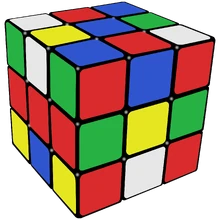11,052 PagesA 3*3*3 Rubik's cube. This picture shows just one out of that number of possible arrangements.

43,252,003,274,489,856,000 is the number of possible legal arrangements of a standard 3×3×3 Rubik's Cube.

This number is a bit over three dozen quintillion.

It is pronounced as follows:

## Approximations

Notation Lower bound Upper bound
Scientific notation $$4.325\times10^{19}$$ $$4.326\times10^{19}$$
Arrow notation $$43\uparrow12$$ $$92\uparrow10$$
Steinhaus-Moser Notation 16 17
Copy notation 3 4
Chained arrow notation $$43\rightarrow12$$ $$92\rightarrow10$$
PlantStar's Debut Notation  
Taro's multivariable Ackermann function A(3,62) A(3,63)
Pound-Star Notation #*(7,0,2)*7 #*(4,0,0,4)*4
BEAF {43,12} {92,10}
Hyper-E notation 4E19 5E19
Bashicu matrix system (0)(0) (0)(0)
Hyperfactorial array notation 20! 21!
Bird's array notation {43,12} {92,10}
Fast-growing hierarchy $$f_2(59)$$ $$f_2(60)$$
Hardy hierarchy $$H_{\omega^2}(59)$$ $$H_{\omega^2}(60)$$
Slow-growing hierarchy $$g_{\omega^{\omega2+2}3+\omega^{\omega2+1}5}(9)$$ $$g_{\omega^{\omega+4}5}(13)$$

## Sources

Community content is available under CC-BY-SA unless otherwise noted.